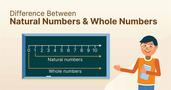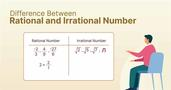Difference Between 2D and 3D Shapes

# Difference Between 2D and 3D Shapes

clickHere
Updated on Nov 9, 2023 17:44 IST

Looking to understand the difference between 2D and 3D shapes? This article offers a comprehensive breakdown of the fundamental differences between these two types of shapes. Whether you’re a beginner or simply looking to refresh your knowledge, this guide has everything you need to know about the basics of 2D and 3D shapes.

Geometry is the branch of mathematics that deals with the study of different shapes and their properties. Shapes (triangles, circles, spheres, cubes, etc.) are fundamental mathematical concepts with various applications in different domains, such as engineering, design, and architecture. In real life, we mainly deal with two types of shapes: 2D and 3D.

This article will explore the difference between 2D and 3D shapes based on different parameters.
So, let’s start!!

Must Check: Top Math Courses for Data Science

Must Check: Top Data Science Online Courses and Certifications

## What is the Difference Between 2D and 3D Shapes?Types of Matrix
Matrix is one of the most important topics of linear algebra that has several applications across the domain. In this article, we will discuss different types of matrix such as...read moreLinear Programming Problem (LPP)
Linear Programming problem or LPP is a method to find the optimum solution of set of parameters that are represented in linear form. In this article, we will discuss all...read moreCorrelation vs Causation
Correlation and causation are one of the most important but confusing topics of statistics. Correlation gives the relationship between two variables, whereas causation means one event is cause due to...read more

## What is a 2D shape?

In geometry, the shape that has only two dimensions are known as 2D shapes. In simple terms, any geometrical shapes with only length and breadth that can be represented on a piece of paper are known as 2D shapes.

Some common 2D shapes are: Triangles, Squares, and Circle

2D shapes are classified into regular and irregular shapes:

Regular Shapes: 2D shapes with equal length sides and equal interior angles are regular shapes.

Example: Square, Equilateral triangle, Pentagon

Irregular Shape: 2D shapes with different sides and angles (interior) are irregular shapes.

Example: Rectangle, Isosceles Triangle

## What is a 3D Shape?

In geometry, shape that has only three dimensions are known as 3D shapes. In simple terms, any geometrical shape with length, breadth, and height.

Dissimilar to 2D shapes, they are difficult to represent on a piece of paper. But these shapes exist in a physical world and can be touched, moved or rotated.

Some common 3D shapes are Spheres, Cubes, Cylinders, and Cones.Transpose of a Matrix
Transpose of a matrix is a matrix flipped over its main diagonal, switching the matrix’s rows and column indices. In this article, we will briefly discuss what transpose of a...read moreTop 10 Features of Python
Python is one of the most popular programming languages nowadays, and Python developers are in extreme demand. It is a flexible programming language that is favored by software engineers and tech organizations...read moreContinuous Variable: How it is Different from Discrete Variable
A numerical variable is said to be a continuous variable if it can take an infinite number of real values within a given interval or range. This article will discuss...read more

## 2d and 3d Shapes Properties

### Properties of 2D Shapes

• Side: Only two sides, made up of straight lines.
• Perimeter: Total length of its sides, calculated by adding all the sides.
• Area: The amount of space any 2d shape takes up, calculated by multiplying length and width.

### Properties of 3D Shapes:

The properties of 3D shapes can be described in terms of their face, edge, vertices, volume, and surface area.

• Faces: 3D shapes have faces made up of flat surfaces that vary depending on the shape.
• Surface Area: It is a sum of the areas of all its faces.
• Volume: Calculated by multiplying length, width, and height.Difference Between Independent and Dependent Variables
Independent variable in mathematics does not depend on another variable and it explains the cause, whereas the dependent variable depends on an independent variable, and it is used to inform...read moreTop 10 Probability Questions Asked in Interviews
Probability is defined as the likeliness of something to occur or happen. In this article, we will discuss top 10 probability questions that are asked in the interviews with their...read moreThe Role of Permutations and Combinations in Data Science
Permutation and combination are one of the most important topics of mathematics that has application in various domain such as machine learning, computer vision etc. In this article, we have...read moreThe Mean Value Theorem in Data Science: A Comprehensive Guide
Mean value theorem or Lagrange mean value theorem states that if f(x) is a continuous and differentiable function over an interval (a, b), then there exists at least one c...read moreDifferential Calculus for Data Science
Differentiation is the process of finding the derivative of a function. The derivative of a function measures the rate of change of a function. In this article, we will discuss...read moreCross Product of two Vectors
Cross product is a binary operation (multiplication) that is performed on two vectors, and the resultant vector is perpendicular to both the given vectors. In this article, we will discuss...read more

## Example of 2D and 3D Shapes

### Example of 3D ShapesStatic vs Dynamic: Understanding the Key Differences for Your Website
A static website contains web pages with fixed content, whereas A dynamic website contains information that changes depending on the viewer, the time of day, the time zone, or the...read moreUnderstanding the Differences Between DDL and DML
DDL commands (statement) is used to create or define the database schema, structure, and constraints, while DML command (or statement) is used to add, retrieve, or update the data, i.e.,...read moreScalars and Vectors: Understanding the Key Differences
Physical quantity with magnitude and no direction is known as a scalar quantity and a physical quantity with magnitude and directions is known as a vector quantity. In this article,...read more

## What are the Key Difference Between 2D Shape and 3D Shapes?

• 2D shapes have only two dimensions (length and width), whereas 3D shapes have three dimensions (length, width, and height).
• 2D shapes can be represented on pieces of paper, while 3D shapes can exist in a physical world that can be touched, moved or removed.
• 3D shapes can have multiple faces, but 2d shapes can have only one.
• 2D shapes can be measured in terms of area, while 3D shapes are measured in terms of surface area and volume.Top 10 Examples of Input and Output Devices
Discover the fascinating world of input and output devices! From keyboards and mice to printers and speakers, this article explores the most common and specialized devices that help us interact...read moreUnderstanding the Basics: The Difference Between Mean, Median, and Mode
Looking to gain a better understanding of statistical measures of central tendency? Check out our article on the differences between mean, median, and mode. Learn how each measure represents a...read moreFrom Zero to One: Understanding the Concept of the Smallest Whole Number

## Conclusion

In this article, we have discussed the differences between 2D and 3D shapes. Hope you will like the article.

Happy Learning!!Unlocking the Mystery of Irrational Numbers: A Guide for Beginners
An irrational number is a number that cannot be expressed as a ratio of two integers. They are infinite and non-repeating decimals that do not terminate, such as pi and...read moreDifference Between Positive and Negative NumbersDifference Between Natural Numbers and Whole NumbersDifference Between Rational and Irrational Number
Want to explore the difference between Rational and Irrational Number or looking for the solution to pi is a rational number or irrational number. Then this blog is for you.Difference Between Module and Package in PythonDifference Between Methods and Functions in Python
In Python, methods and functions have similar purposes but differ in important ways. Functions are independent blocks of code that can be called from anywhere, while methods are tied to...read more

## FAQs

What is a 2D shape?

A 2D shape is a flat, two-dimensional object that only has length and width, such as a square or a circle.

What is a 3D shape?

A 3D shape is a solid, three-dimensional object that has length, width, and depth, such as a cube or a sphere.

What is the main difference between 2D and 3D shapes?

The main difference between 2D and 3D shapes is that 2D shapes are flat and have only two dimensions (length and width), whereas 3D shapes are solid and have three dimensions (length, width, and depth).

How can you identify a 2D shape?

2D shape can be identified by looking for its defining features of length and width. Examples of 2D shapes include squares, rectangles, and triangles.

How can you identify a 3D shape?

3D shape can be identified by looking for its defining features of length, width, and depth. Examples of 3D shapes include cubes, spheres, and pyramids.

clickHere

Vikram has a Postgraduate degree in Applied Mathematics, with a keen interest in Data Science and Machine Learning. He has experience of 2+ years in content creation in Mathematics, Statistics, Data Science, and Mac... Read Full Bio

## Trending Data Science CoursesBecome a Data Science Professional with IIT Certification in Advanced ProgrammingBecome a Data Science Professional with IIT Certif...
Guvi5.0Machine Learning
Coursera4.6Python Certificate
IIT Madras4.4Postgraduate Program in Data Science with Generative AIPostgraduate Program in Data Science with Generati...
Praxis Tech School4.8Starts 5 DecBecome a Data Science Professional with IIT Certification in Advanced ProgrammingBecome a Data Science Professional with IIT Certif...
Guvi5.0Starts 15 Dec

## Top Picks & New Arrivals